7 Determination of the Net Business Rate

For the formulation of the net business ratewe need a statement about the ratio of investment into the real economy to the transactions of the financial system to itself, that means the relation between direct and indirect investment. Let us consider again the differential equation of capital: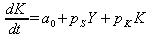. The coefficient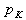is the effective interest on the capital this year. This interest stems from the two components of direct and indirect use of capital. So we can split to: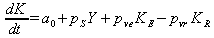(7.1)

Because the share of capital stock which is sold within the financial industry immediately gains returns. The proportion which is given initially in the real economy reduces the capital stock this year and receives its return later, presumable next year. The interest rates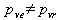however can also be different. With the simple substitutiowe get now: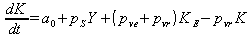(7.2)

So we have to carry two blocks from the income and expenditure which is the overall result. But we can summarize this flat in one rate by(7.3)

defined as the difference between the results of expenditure and revenue. Withthen as the average nominal interest rate on all types of assets. And the proportion who fail this year due to the credit of it is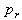. This approach simplifies calculations thereafter significantly. Next we define the relative value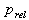as the share which represents the direct use of capital to total capital stock: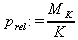(7.4)

This value was in 1950, two years after the introduction of the German mark, according to figures from the Bundesbank14 at about 73% and decreased continuously to less than 40% in 2010. This can be phenomenologically explained by the fact that at the beginning15 of an economy the capital is available to virtually all loans (investment and consumptive) as flows into the real economy. With increasing time and thus increasing capital stock, more and more money goes into the banks own business (the "Investment Banking"), as the yield-oriented investments get more and more scarce in the real economy over time.

Since we now have to specify the relative proportion not absolute, but as a percentage of total interest income in our equation, we write this value to something different:is the share of interest incomethat comes from lending into the real economy as an absolute part of a percentage. Thus applies to the net business ratio16after the reinvestment ofto the GDP now in terms ofas: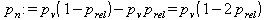(7.5)

If we look at the real data of(Fig. 4), then we see its regular course. We can start with a simple phenomenological17 approximation, namely that the share of reinvestment decreases slowly according to a simple exponential form: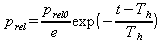(7.6)

As is easily seen, this function starts () with the value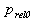. After the exponential half-lifetime18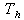it falls off to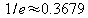and then very slowly goes towards zero. Our formula is therefore justified phenomenologically as(7.7)    withaverage nominal interest rate over all assets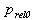Initial direct capital investment as a share of total capital stock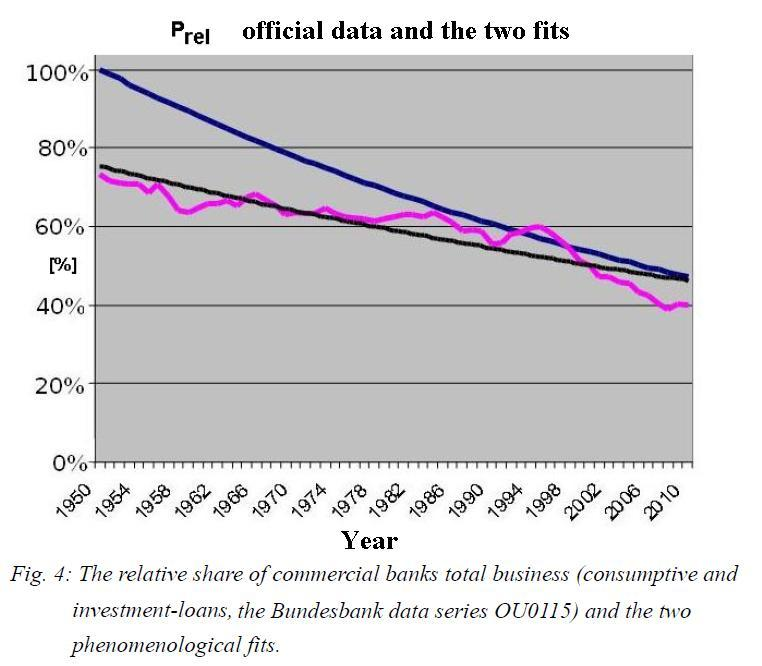The period after the direct capital investment has dropped to about 1/e=36.7% (exponential half-lifetime). In order to eliminate the specific initial parameter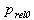and in the interest of generality and a purely phenomenological analytical consideration, it can be assumed that at the beginning of an economy almost 100% of the capital is used for the loans to finance the real economy (upper fit curve).